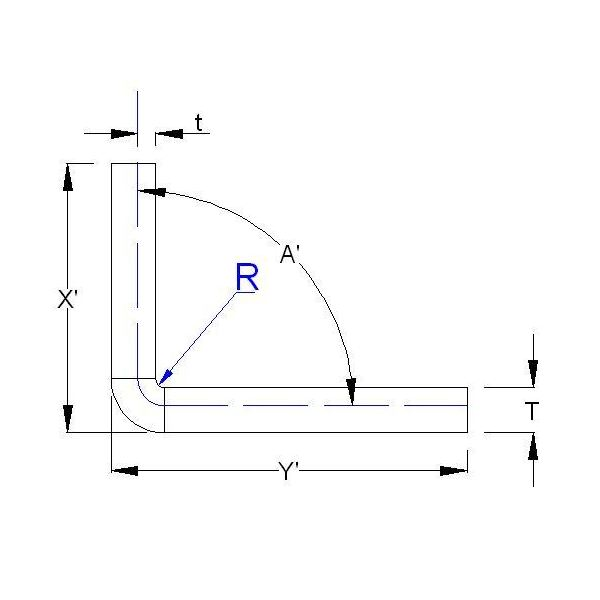# Sheet Metal Design Guide: Calculate Bending Allowance Accurately

Page content

## Typical Sheet Metal Bending Process

Sheet metal bending process can be briefly jotted down as:

• Developed sheet metal size is obtained from drawing.
• Developed sized sheet metal is cut out from large sheet by punching operation.
• Bending brake is used to bend the sheet metal piece to the required shape and angle.

## Bending Allowance Calculation

Bending allowance is the input for calculating developed sheet metal size. How? See the snap below:

If you have a sheet metal bending product (as shown in above picture) with two legs of length “X” and “Y” and you unbend it, you will see that total length of the unbend sheet will NOT X + Y rather, the length will be X + Y + BA. Where, BA is bending allowance.

The formula for calculating sheet metal bending allowance is (Please refer to the above picture):

BA= Bend Angle * (Π/180)* (R+ K factor* T)……Eqn.1

Where,

BA is Bend allowance.

Bend Angle represents the angle to which sheet metal has bend (here it is 90 degree).

R is inner bend radius.

K factor= t/T……….Eqn.2

So, if you got K factor value now, you can easily calculate bending allowance.

## Calculating K factor

There are standard charts available with sheet metal design handbook for calculating K factor (ideally it should be 0.5). But the most accurate way to find out K factor is reverse engineering of a small but same sheet metal sample piece. Procedure is as below:• Now by referring Fig.1 and Fig.2 we can write:

X= X’ - (tan (A’/2))*(T+R)…..Eqn.3

Y=Y’- (tan (A’/2))*(T+R)……Eqn.4

Since, all the items of right hand sides of the Eqn.3 and Eqn.4 are known so we can get the values of X and Y of the sample.

• We also know the developed length of the sample (P).
• By doing P – (X+Y), we can get the bend allowance (BA) for the sample.
• Now, use Eqn.1 and you will get K factor. This K factor will be same for the actual product.
• Use this K factor value to calculate bending allowance for main product.

## Conclusion

For getting accurate output of sheet metal bending process you need to calculate accurate sheet metal developed length. And for calculating accurate sheet metal developed length you need to accurately calculate sheet metal bending allowance and for bending allowance calculation you need to have accurate K factor value. The procedure discussed in this sheet metal design guide is the simplest way to calculate bend allowance with accepted accuracy.

• Creating die and punch for sheet metal parts using pro engineer: Pro Engineer is developed in such a way that the feature operations in ProE are actually similar to real life manufacturing operations. Form feature in Pro Engineer is identical to the real life sheet metal forming operations (where components are produced by sheet metal punch and Die press tools.
• Overview of Sheet Metal Design using Pro Engineer: Pro engineer is considered to be the leading software for sheet metal design. Like surfacing of catia, sheet metal design module of pro engineer is the unique selling feature of the software